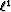Next: Principles of norm and Up: Adaptive subtraction of multiples Previous: Summary

# Introduction

A classical approach for attenuating multiples consists of building a multiple model, e.g., as described by Verschuur et al., 1992 or Berkhout and Verschuur 1997, and adaptively subtracting this model from the multiple infested-data by estimating shaping filters Dragoset (1995); Liu et al. (2000); Rickett et al. (2001). The estimation of the shaping filters is usually done in a least-squares sense making these filters relatively easy to compute. Implicitly, by using thenorm, the resulting signal, after the filter estimation step, is assumed to be orthogonal to the noise and has minimum energy. These assumptions might not hold and other methods, such as pattern-based approaches Guitton et al. (2001); Spitz (1999) have been proposed to circumvent these limitations. For instance, when a strong primary is surrounded by weaker multiples, the multiple model will match the noise (multiples) as well as the signal (primaries) such that the difference between the data and the filtered multiple model is minimum in a least-squares sense. Consequently, some primary energy might leak in the estimated multiples and vice versa. Therefore, a new criterion or norm for the filter estimation step is needed.

In this paper, I propose estimating the shaping filters with thenorm instead of thenorm, thus relaxing the need for the signal to have minimum energy. This choice is driven by the simple fact that thenorm is robust to outliers'' Claerbout and Muir (1973) and large amplitude anomalies. Because thenorm is singular where any residual component vanishes, the Huber norm with L-BFGS solver of Chapteris chosen instead. This method gives an excellent approximation of thenorm.

In this first section following the introduction, some limitations of the least-squares criterion on a simple 1-D problem are illustrated. I then introduce the proposed approach based on thenorm to improve the multiple attenuation results. In a second synthetic example, internal multiples are attenuated with theandnorms. Finally, I utilize shaping filters on a multiple contaminated gather from a seismic survey showing that thenorm leads to a substantial attenuation of the multiples.Next: Principles of norm and Up: Adaptive subtraction of multiples Previous: Summary
Stanford Exploration Project
5/5/2005